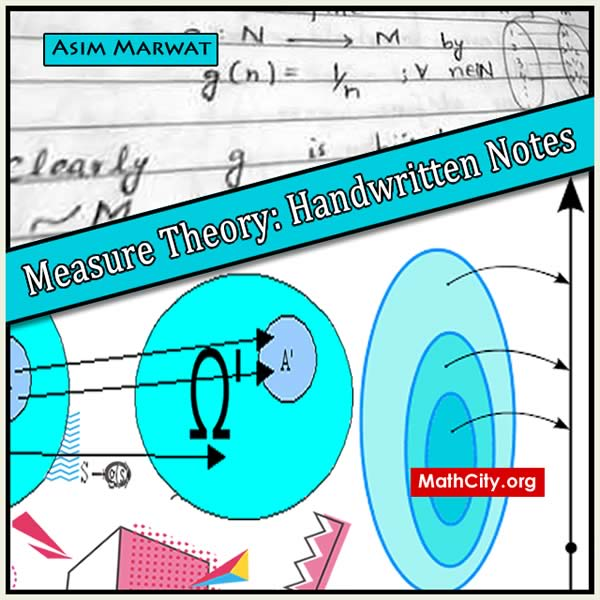# Measure Theory Handwritten Notes by Asim MarwatThese notes are made and shared by Mr. Asim Marwat. He has our sincere gratitude for supplying these notes, and we value his effort in having them published on MathCity.org. Measure Theory is an important subject in BS Mathematics. These notes contain topic from base level, like equivalence set, to advance level, like convergent in measure.

 Name Measure Theory: Handwritten Notes Mr. Asim Marwat 247 pages PDF (see Software section for PDF Reader) 14.7 MB
• Equivalent set
• Infinite set
• Denumerable set, Non-denumerable set
• Countable set
• Semi ring, Sigma set, Algebra
• Measure, Measure Space
• Outer Measure (Carathedory Measure)
• Measurable set, Null set
• Lebesgue Measure
• Zermelo's Axiom, Almost Everywhere
• Measurable Function
• Limit Superior of a Sequence
• Limit Inferior of a Sequence
• Limit Superior, Limit Inferior
• Characteristic Function, Simple Function
• Lebesgue Integral of Simple Function
• Tchebeshev's Inequality
• Monotone Convergence Theorem
• Fatou's Lema
• Lebesgue Dominated Convergence Theorem for Non-negative Measurable Function
• Convergence Theorem
• Monoton Convergence Theorem
• Lebesgue Dominated Convergence Theorem
• Lebesgue Bounded Convergence Theorem
• Comparison Between Lebesgue and Riemann Integral
• Lebesgue Integral
• Uniform Convergence Theorem
• Lebesgue Integral for Unbounded Function
• Lebesgue Integral for arbitrary Unbounded Function
• $L_p$ Space, $L_p$ as Normed Linear Space
• Holder Inequality
• Minkowski's Inequality
• Essential Bounded
• Minkowski's Inequality for $L^\infty$
• Convergence in $L_p$ or Mean Convergence
• Convergent in Measure

Please click on View Online to see inside the PDF.